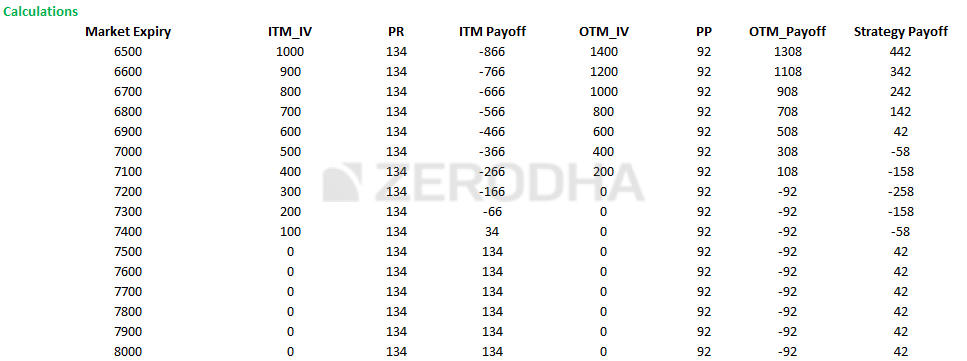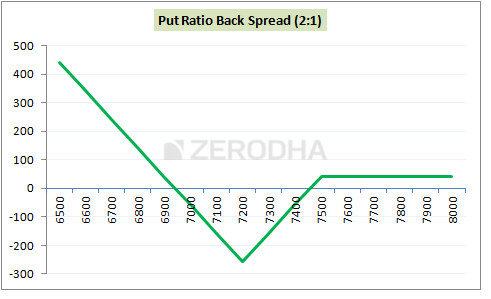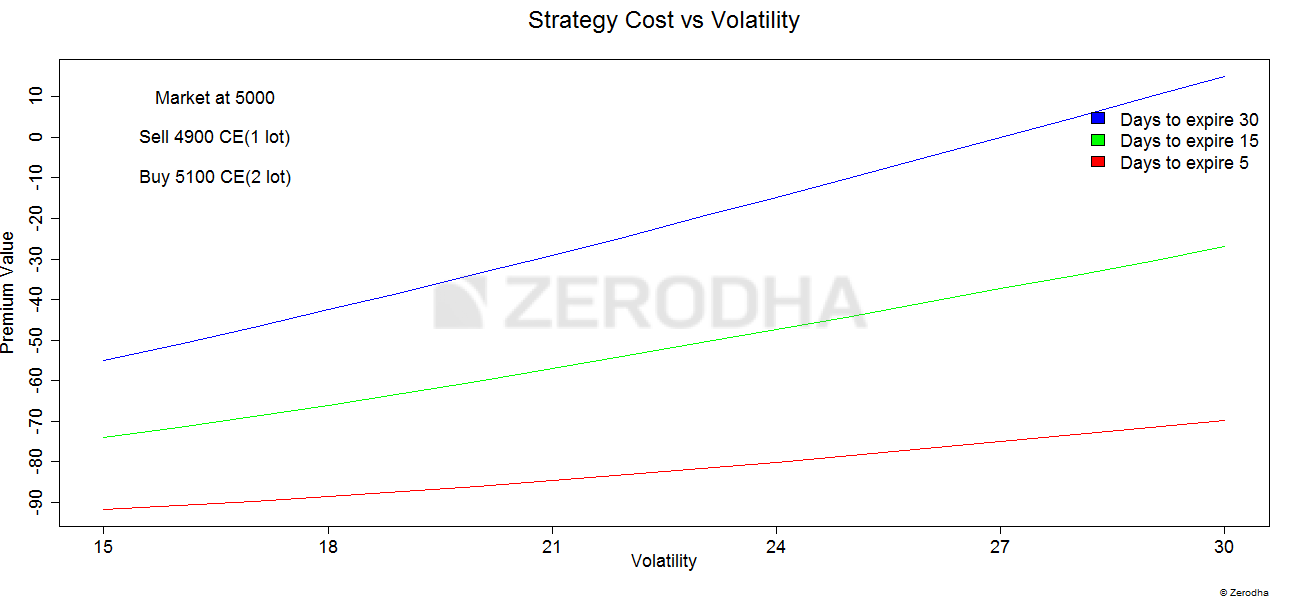# 9. Put Ratio Back spread

## 9.1 – Background

We discussed the “Call Ratio Back spread” strategy extensively in chapter 4 of this module. The Put ratio back spread is similar except that the trader invokes this when he is bearish on the market or stock.

At a broad level this is what you will experience when you implement the Put Ratio Back Spread

1. Unlimited profit if the market goes down
2. Limited profit if market goes up
3. A predefined loss if the market stays within a range

In simpler words you make money as long as the market moves in either direction, of course the strategy is more favorable if market goes down.Usually, the Put Ratio Back Spread is deployed for a ‘net credit’, meaning money flows into your account as soon as you execute Put Ratio Back Spread. The ‘net credit’ is what you make if the market goes up, as opposed to your expectation (i.e market going down). On the other hand if the market indeed goes down, then you stand to make an unlimited profit.

I suppose this should also explain why the put ratio back spread is better than buying a plain vanilla put option.

## 9.2 – Strategy Notes

The Put Ratio Back Spread is a 3 leg option strategy as it involves buying two OTM Put options and selling one ITM Put option. This is the classic 2:1 combo. In fact the put ratio back spread has to be executed in the 2:1 ratio meaning 2 options bought for every one option sold, or 3 options bought for every 2 options sold, so on and so forth.

Let take an example – Nifty Spot is at 7506 and you expect Nifty to hit 7000 by the end of expiry. This is clearly a bearish expectation. To implement the Put Ratio Back Spread –

1. Sell one lot of 7500 PE (ITM)
2. Buy two lots of 7200 PE (OTM)

Make sure –

1. The Put options belong to the same expiry
2. Belong to the same underlying
3. The ratio is maintained

The trade set up looks like this –

1. 7500 PE, one lot short, the premium received for this is Rs.134/-
2. 7200 PE, two lots long, the premium paid is Rs.46/- per lot, so Rs.92/- for 2 lots
3. Net Cash flow is = Premium Received – Premium Paid i.e 134 – 92 = 42 (Net Credit)

With these trades, the Put ratio back spread is executed. Let us check what would happen to the overall cash flow of the strategies at different levels of expiry.

Do note we need to evaluate the strategy payoff at various levels of expiry, as the strategy payoff is quite versatile.

Scenario 1 – Market expires at 7600 (above the ITM option)

At 7600, both the Put options would expire worthless. The intrinsic value of options and the eventual strategy payoff is as below –

• 7200 PE, would expire worthless, since we are long 2 lots of this option at Rs.46 per lot, we would lose the entire premium of Rs.92 paid
• 7500 PE would also expire worthless, but we have written this option and received a premium of Rs.134, which in this case can be retained back
• The net payoff from the strategy is 134 – 92 = 42

Do note, the net payoff of the strategy at 7600 (higher than the ITM strike) is equivalent to the net credit.

Scenario 2 – Market expires at 7500 (at the higher strike i.e the ITM option)

At 7500 both the options would have no intrinsic value, hence they both would expire worthless. Hence the payoff would be similar to the payoff we discussed at 7600. Hence the net strategy payoff would be equal to Rs.42 (net credit).

In fact as you may have guessed, the payoff of the strategy at any point above 7500 is equal to the net credit.

Scenario 3 – Market expires at 7458 (higher break even)

Like in the call ratio back spread strategy, the put ratio back spread too has two breakeven points i.e the upper breakeven and the lower breakeven point. 7458 marks the upper breakeven level; of course we will discuss how we arrived at the upper breakeven point a little later in the chapter.

• At 7458, the 7500 PE will have an intrinsic value. As you may recall, the put option intrinsic value can be calculated as Max[Strike – Spot, 0] i.e Max[7500 – 7458, 0] hence 42
• Since we have sold 7500 PE at 134, we will lose a portion of the premium received and retain the rest. Hence the payoff would be 134 – 42 = 92
• The 7200 PE will not have any intrinsic value, hence the entire premium paid i.e 92 is lost
• So on one hand we made 92 on the 7500 PE and on the other we would lose 92 on the 7200 PE resulting in no loss, no gain. Thus, 7458 marks as one of the breakeven points.

Scenario 4 – Market expires at 7200 (Point of maximum pain)

This is the point at which the strategy causes maximum pain, let us figure out why.

• At 7200, 7500 PE would have an intrinsic value of 300 (7500 – 7200). Since we have sold this option and received a premium of Rs.134, we would lose the entire premium received and more. The payoff on this would be 134 – 300 = – 166
• 7200 PE would expire worthless as it has no intrinsic value. Hence the entire premium paid of Rs.92 would be lost
• The net strategy payoff would be -166 – 92 = – 258
• This is a point where both the options would turn against us, hence is considered as the point of maximum pain

Scenario 5 – Market expires at 6942 (lower break even)

At 6942, both the options would have an intrinsic value; however this is the lower breakeven point. Let’s figure out how this works –

• At 6942, 7500 PE will have an intrinsic value equivalent of 7500 – 6942 = 558. Since have sold this option at 134, the payoff would be 134 – 558 = – 424
• The 7200 PE will also have an intrinsic value equivalent of 7200 – 6942 = 258 per lot, since we are long two lots the intrinsic value adds upto 516. We have initially paid a premium of Rs.92 (both lots included), hence this needs to be deducted to arrive at the payoff would be 516 – 92 = +424
• So on one hand we make 424 on the 7200 PE and on the other we would lose 424 on the 7500 PE resulting in no loss, no gain. Thus, 6942 marks as one of the breakeven points.

Scenario 6 – Market expires at 6800 (below the lower strike price)

Remember, the put ratio backspread is a bearish strategy. It is supposed to make money once the market goes below the lower breakeven point. So lets understand how the pay off behaves at a point lower than the lower breakeven point.

• At 6800, 7500 PE will have an intrinsic value of 700 and since we are short 7500PE at 134, we would lose 134 -700 = – 566
• 7200 PE will have an intrinsic value of 400. Since we are long 2 lots, the intrinsic value would be 800. Premium paid for two lots is Rs.92, hence after adjusting for the premium paid, we get to make 800 – 92 = +708
• Net strategy payoff would be 708 – 566 = +142

Likewise, you can evaluate the strategy payoff at different levels of market expiry and you will realize that the profits are uncapped as long as the market continues to slide. The following table showcases the same –Plotting the different payoff points, gives us the strategy payoff graph –Clearly from the graph above, we can conclude –

1. If markets go down, then the profits are unlimited
2. There are two breakeven points
3. The point at which maximum loss occurs is at 7200
4. If markets goes up, then the profits are limited

## 9.3 – Strategy generalization

We can generalize the key strategy levels as below –

1. Spread = Higher Strike – lower strike
1. 7500 – 7200 = 300
2. Max loss = Spread – Net credit
1. 300 – 42 = 258
3. Max Loss occurs at = Lower strike price
4. Lower Breakeven point = Lower strike – Max loss
1. 7200 – 258 = 6942
5. Upper breakeven point = Lower strike + Max loss
1. 7200 + 258 = 7458

## 9.4 – Delta, strike selection, and effect of volatility

As we know, the strategy gets more profitable as and when the market falls. In other words this is a directional strategy (profitable when markets go down) and therefore the delta at overall strategy level should reflect this. Let us do the math to figure this out –

• 7500 PE is ITM option, delta is – 0.55. However since we have written the option, the delta is –(-0.55) = +0.55
• 7200 PE is OTM, has a delta of – 0.29, remember we are long two lots here
• The overall position delta would be +0.55 + (-0.29) +(-0.29) = – 0.03

The non zero Delta value clearly indicates that the strategy is sensitive to the directional movement (although negligible). The negative sign indicates that the strategy makes money when the market goes down.

As far as the strikes are concerned, I’d suggest you stick to the classic combination of ITM and OTM options. Remember the trade needs to be executed for a ‘Net Credit’. Do not initiate this strategy if there is a net outflow of cash at the time of execution.

Let’s look at the variation in volatility and its effect on the strategy –There are three colored lines depicting the change of “premium value” versus change in volatility. These lines help us understand the effect of increase in volatility on the strategy keeping time to expiry in perspective.

1. Blue Line – This line suggests that an increase in volatility when there is ample time to expiry (30 days) is beneficial for the Put ratio back spread. As we can see the strategy payoff increases from -57 to +10 when the volatility increase from 15% to 30%. Clearly this means that when there is ample time to expiry, besides being right on the direction of stock/index you also need to have a view on volatility. For this reason, even though I’m bearish on the stock, I would be a bit hesitant to deploy this strategy at the start of the series if the volatility is on the higher side (say more than double of the usual volatility reading)
2. Green line – This line suggests that an increase in volatility when there are about 15 days time to expiry is beneficial, although not as much as in the previous case. As we can see the strategy payoff increases from -77 to -47 when the volatility increase from 15% to 30%.
3. Red line – Clearly increase in volatility when we have a few days to expiry does not have much impact on the premium value. This means, when you are close to expiry you only need to worry about the directional movement and need not really worry much about the variation in volatility.

### Key takeaways from this chapter

1. The Put Ratio Back spread is best executed when your outlook on the stock/index is bearish
2. The strategy requires you to sell 1 ITM PE and buy 2 OTM PE, and this is to be executed in the same ratio i.e for every 1 option sold, 2 options have to be purchased
3. The strategy is usually executed for a ‘Net Credit’
4. The strategy makes limited money if the stock price goes up, and unlimited profit when the stock price goes down
5. There are two break even points – lower breakeven and upper breakeven
6. Spread = Higher Strike – Lower Strike
8. Max Loss = Spread – Net Credit
9. Max Loss occurs at = Lower Strike
10. The payoff when market goes up = Net Credit
11. Lower Breakeven = Lower Strike – Max Loss
12. Upper Breakeven = Lower Strike + Max Loss
13. Irrespective of the time to expiry opt for ITM and OTM strike combination
14. Increase in volatility is good for this strategy when there is more time to expiry

1.Shrekanth says:

Very useful topic. Plz clear my small doubt. Can we executive a call ratio back spread or the put ratio back spread on net debit? Do zerodha allows that? Kindly clarify.

•Karthik Rangappa says:

You can execute it for a net debit…for example if you sell ATM and buy a strike just next to ATM then it ‘may’ result in a net debit. However its best if you can execute it for a next credit. You can do this with any broker, not just Zerodha.

•Shrekanth says:

Thanks for the prompt reply Karthik. It’s clear now. If I have to impliment the ratio spreads with an intraday or 1-2 days view, what sort of monyness you recommend for the option strike price.

•Karthik Rangappa says:

I would not suggest you do this for intraday. However if you wish to set this up overnight, I’d suggest you look at the standard combo – ATM and OTM.

•SANJAY says:

a VERY tricky question regards to Hedging which time and strike price would be the best in bank nifty or nifty for doing hedging regardless volatility in the market. also how could we identify trends in the market like bullish or bearish trend?

•Karthik Rangappa says:

This comes purely based on experience, Sanjay 🙂

2.chandra says:

sir, i am new trader, i read your articles. All are too good. but i am not having that much time now to concentrate on market. is there any paid service to give successful traders ideas or calls

•Karthik Rangappa says:

You should check out – https://opentrade.in/

3.Madhujeet says:

Do we need to make the delta neutral while implementing this strategy and suppose if we put this strategy then can we make adjustments by keeping delta neutral after respective time interval???

•Karthik Rangappa says:

By its structure, the strategy will remain delta neutral.

4.amit gupta says:

please confirm while sell ITM and Buy OTM must be done in a time frame,or i can buy PE 2 lot OTM and sell ITM some other day or few days later like after 7 days ,then whether this strategy will work .and what should be ITM strike position along with volatility .

•Karthik Rangappa says:

It has to be executed as a set in one shot, you cannot spit it over time.

5.sandeep says:

in this strategy as expected if volatility and movement does not come than @ what point should we exit the trade

•Karthik Rangappa says:

You can hold the position till expiry…but if the position is making you uncomfortable, then you should exit.